# SBI PO Prelims 2022 Quantitative Aptitude Quiz- 8

## SBI PO Prelims 2022 Quantitative Aptitude Quiz

Quantitative Aptitude is the most significant part of almost all competitive exams. Many candidates find difficulty in solving Quantitative Aptitude Questions. The only way to ace this section is to practice as many questions as possible. And, to help you practice more and more questions, we have come up with the SBI PO Prelims Quantitative Aptitude Quiz. This SBI PO Prelims Quantitative Aptitude Quiz is completely Free. This SBI PO Prelims Quantitative Aptitude Quiz includes a variety of questions that are usually asked in preliminary and main exams of various banking exams. Candidates will be provided with a detailed solution for each question in this SBI PO Prelims Quantitative Aptitude Quiz. This SBI PO Prelims Quantitative Aptitude Quiz will assist candidates in better preparing for upcoming exams.

Directions (1-5): In each of these questions, two equations (I) and (II) are given. You have to solve both the equations and give answer.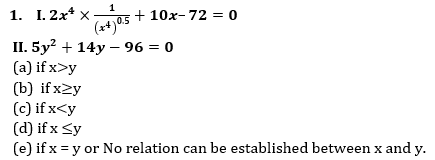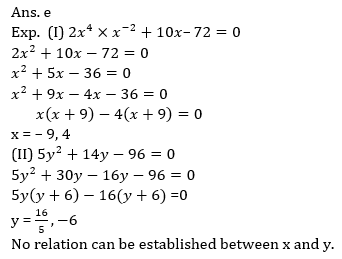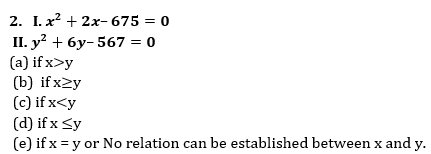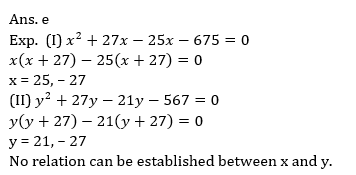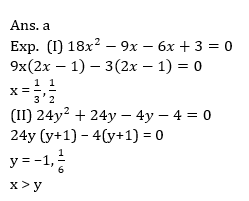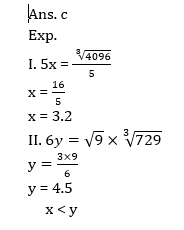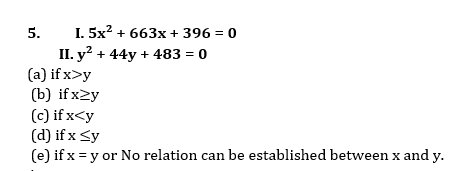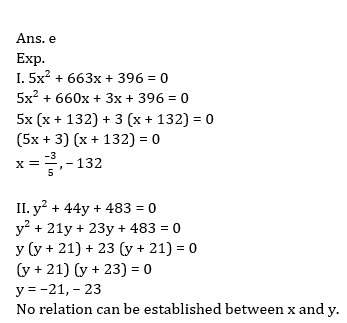Directions (6-10): The following questions are accompanied by two statements (i) and (ii). You have to determine which statement(s) is/are necessary/sufficient to answer the question.

6. In a group of boys and girls, 20% boys and 30% girls like the game chess. Find the total number of boys in the group.
(i) Number of boys like chess is equal to the number of girls like chess.
(ii) 70 girls present in the group do not like chess.
(a) Only (i)
(b) Only (ii)
(c) Only (i) or Only (ii).
(d) Both (i) and (ii) together required.
(e) Both (i) and (ii) are not sufficient7. Find the probability of getting one rotten egg.
(i) Probability of getting 2 rotten egg from the total of 10 egg is 2/15.
(ii) Number of rotten egg is 2 less than the number of fresh egg.
(a) Only (i)
(b) Only (ii)
(c) Only (i) or Only (ii)
(d) Both (i) and (ii) together required.
(e) Both (i) and (ii) are not sufficient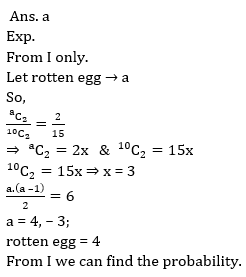8. Find the rate of interest.
(i) A sum invested on S.I. for 5 year becomes Rs5160.
(ii) A sum become 4 times after 2 years on C.I
(a) Only (i)
(b) Only (ii)
(c) Only (i) or Only (ii)
(d) Both (i) and (ii) together required.
(e) Both (i) and (ii) are not sufficient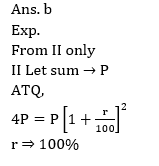9. A quadrilateral ABCD is drawn to circumscribe a circle having center O. Find the length of AB(i) length of BC and CR is given
(ii) Radius of circle and length of OA is given.
(a) Only (i)
(b) Only (ii)
(c) Only (i) or Only (ii)
(d) Both (i) and (ii) together required.
(e) Both (i) and (ii) are not sufficient10. Find the profit on selling an article?
(i) It is found that profit calculated on selling price is 20%.
(ii) Article is marked 50% above cost price and a discount of 20% is given on it. If 5% less discount is given, then he could have earn Rs.75.6 more.
(a) Only (i)
(b) Only (ii)
(c) Only (i) or Only (ii)
(d) Both (i) and (ii) together required.
(e) Both (i) and (ii) are not sufficient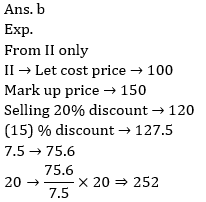###Click to Buy Bank MahaCombo Package

Recommended PDF’s for:

#### Most important PDF’s for Bank, SSC, Railway and Other Government Exam : Download PDF Now

AATMA-NIRBHAR Series- Static GK/Awareness Practice Ebook PDF Get PDF here
The Banking Awareness 500 MCQs E-book| Bilingual (Hindi + English) Get PDF here
AATMA-NIRBHAR Series- Banking Awareness Practice Ebook PDF Get PDF here
Computer Awareness Capsule 2.O Get PDF here
AATMA-NIRBHAR Series Quantitative Aptitude Topic-Wise PDF Get PDF here
AATMA-NIRBHAR Series Reasoning Topic-Wise PDF Get PDF Here
Memory Based Puzzle E-book | 2016-19 Exams Covered Get PDF here
Caselet Data Interpretation 200 Questions Get PDF here
Puzzle & Seating Arrangement E-Book for BANK PO MAINS (Vol-1) Get PDF here
ARITHMETIC DATA INTERPRETATION 2.O E-book Get PDF here

3